# Excel logical operators: equal to, not equal to, greater than, less than

Many tasks you perform in Excel involve comparing data in different cells. For this, Microsoft Excel provides six logical operators, which are also called comparison operators. This tutorial aims to help you understand the insight of Excel logical operators and write the most efficient formulas for your data analysis.

## Excel logical operators - overview

A logical operator is used in Excel to compare two values. Logical operators are sometimes called Boolean operators because the result of the comparison in any given case can only be either TRUE or FALSE.

Six logical operators are available in Excel. The following table explains what each of them does and illustrates the theory with formula examples.

 Condition Operator Formula Example Description Equal to = =A1=B1 The formula returns TRUE if a value in cell A1 is equal to the values in cell B1; FALSE otherwise. Not equal to <> =A1<>B1 The formula returns TRUE if a value in cell A1 is not equal to the value in cell B1; FALSE otherwise. Greater than > =A1>B1 The formula returns TRUE if a value in cell A1 is greater than a value in cell B1; otherwise it returns FALSE. Less than < =A1= =A1>=B1 The formula returns TRUE if a value in cell A1 is greater than or equal to the values in cell B1; FALSE otherwise. Less than or equal to <= =A1<=B1 The formula returns TRUE if a value in cell A1 is less than or equal to the values in cell B1; FALSE otherwise.

The screenshot below demonstrates the results returned by Equal to, Not equal to, Greater than and Less than logical operators: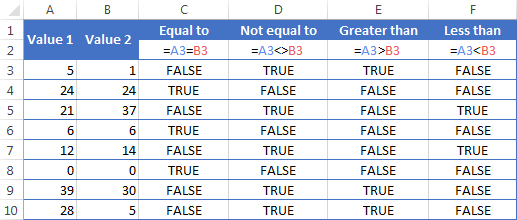It may seem that the above table covers it all and there's nothing more to talk about. But in fact, each logical operator has its own specificities and knowing them can help you harness the real power of Excel formulas.

### Using "Equal to" logical operator in Excel

The Equal to logical operator (=) can be used to compare all data types - numbers, dates, text values, Booleans, as well as the results returned by other Excel formulas. For example:

 =A1=B1 Returns TRUE if the values in cells A1 and B1 are the same, FALSE otherwise. =A1="oranges" Returns TRUE if cells A1 contain the word "oranges", FALSE otherwise. =A1=TRUE Returns TRUE if cells A1 contain the Boolean value TRUE, otherwise it returns FALSE. =A1=(B1/2) Returns TRUE if a number in cell A1 is equal to the quotient of the division of B1 by 2, FALSE otherwise.

#### Example 1. Using the "Equal to" operator with dates

You might be surprised to know that the Equal to logical operator cannot compare dates as easily as numbers. For example, if the cells A1 and A2 contain the date "12/1/2014", the formula `=A1=A2` will return TRUE exactly as it should.

However, if you try either `=A1=12/1/2014` or `=A1="12/1/2014"` you will get FALSE as the result. A bit unexpected, eh?

The point is that Excel stores dates as numbers beginning with 1-Jan-1900, which is stored as 1. The date 12/1/2014 is stored as 41974. In the above formulas, Microsoft Excel interprets "12/1/2014" as a usual text string, and since "12/1/2014" is not equal to 41974, it returns FALSE.

To get the correct result, you must always wrap a date in the DATEVALUE function, like this `=A1=DATEVALUE("12/1/2014")`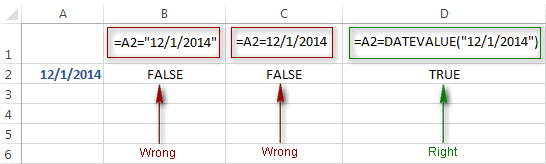Note. The DATEVALUE function needs to be used with other logical operator as well, as demonstrated in the examples that follow.

The same approach should be applied when you use Excel's equal to operator in the logical test of the IF function. You can find more info as well as a few formula examples in this tutorial: Using Excel IF function with dates.

#### Example 2. Using the "Equal to" operator with text values

Using Excel's Equal to operator with text values does not require any extra twists. The only thing you should keep in mind is that the Equal to logical operator in Excel is case-insensitive, meaning that case differences are ignored when comparing text values.

For example, if cell A1 contains the word "oranges" and cell B1 contains "Oranges", the formula `=A1=B1` will return TRUE.

If you want to compare text values taking in to account their case differences, you should use the EXACT function instead of the Equal to operator. The syntax of the EXACT function is as simple as:

EXACT(text1, text2)

Where text 1 and text2 are the values you want to compare. If the values are exactly the same, including case, Excel returns TRUE; otherwise, it returns FALSE. You can also use the EXACT function in IF formulas when you need a case-sensitive comparison of text values, as shown in the below screenshot: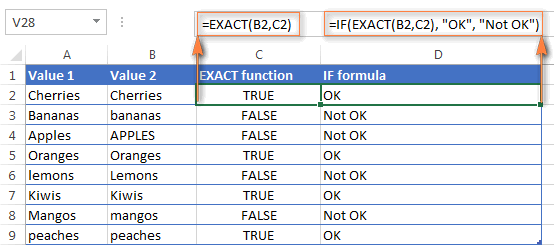Note. If you want to compare the length of two text values, you can use the LEN function instead, for example `=LEN(A2)=LEN(B2)` or `=LEN(A2)>=LEN(B2)`.

#### Example 3. Comparing Boolean values and numbers

There is a widespread opinion that in Microsoft Excel the Boolean value of TRUE always equates to 1 and FALSE to 0. However, this is only partially true, and the key word here is "always" or more precisely "not always" : )

When writing an 'equal to' logical expression that compares a Boolean value and a number, you need to specifically point out for Excel that a non-numeric Boolean value should be treated as a number. You can do this by adding the double minus sign in front of a Boolean value or a cell reference, e. g. `=A2=--TRUE` or `=A2=--B2`.

The 1st minus sign, which is technically called the unary operator, coerces TRUE/FALSE to -1/0, respectively, and the second unary negates the values turning them into +1 and 0. This will probably be easier to understand looking at the following screenshot: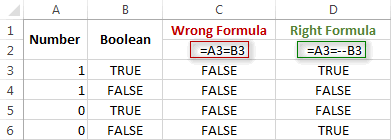Note. You should add the double unary operator before a Boolean when using other logical operators such as not equal to, greater than or less than to correctly compare a numeric and Boolean values.

When using logical operators in complex formulas, you might also need to add the double unary before each logical expression that returns TRUE or FALSE as the result. Here's an example of such a formula: SUMPRODUCT and SUMIFS in Excel.

### Using "Not equal to" logical operator in Excel

You use Excel's Not equal to operator (<>) when you want to make sure that a cell's value is not equal to a specified value. The use of the Not equal to operator is very similar to the use of Equal to that we discussed a moment ago.

The results returned by the Not equal to operator are analogous to the results produced by the Excel NOT function that reverses the value of its argument. The following table provides a few formula examples.

 Not equal to operator NOT function Description =A1<>B1 =NOT(A1=B1) Returns TRUE if the values in cells A1 and B1 are not the same, FALSE otherwise. =A1<>"oranges" =NOT(A1="oranges") Returns TRUE if cell A1 contains any value other than "oranges", FALSE if it contains "oranges" or "ORANGES" or "Oranges", etc. =A1<>TRUE =NOT(A1=TRUE) Returns TRUE if cell A1 contains any value other than TRUE, FALSE otherwise. =A1<>(B1/2) =NOT(A1=B1/2) Returns TRUE if a number in cell A1 is not equal to the quotient of the division of B1 by 2, FALSE otherwise. =A1<>DATEVALUE("12/1/2014") =NOT(A1=DATEVALUE("12/1/2014")) Returns TRUE if A1 contains any value other than the date of 1-Dec-2014, regardless of the date format, FALSE otherwise.

### Greater than, less than, greater than or equal to, less than or equal to

You use these logical operators in Excel to check how one number compares to another. Microsoft Excel provides 4 comparison operates whose names are self-explanatory:

• Greater than (>)
• Greater than or equal to (>=)
• Less than (<)
• Less than or equal to (<=)

Most often, Excel comparison operators are used with numbers, date and time values. For example:

 =A1>20 Returns TRUE if a number in cell A1 is greater than 20, FALSE otherwise. =A1>=(B1/2) Returns TRUE if a number in cell A1 is greater than or equal to the quotient of the division of B1 by 2, FALSE otherwise. =A1

#### Using Excel comparison operators with text values

In theory, you can also use the greater than, greater than or equal to operators as well as their less than counterparts with text values. For example, if cell A1 contains "apples" and B1 contains "bananas", guess what the formula `=A1>B1` will return? Congratulations to those who've staked on FALSE : )

When comparing text values, Microsoft Excel ignores their case and compares the values symbol by symbol, "a" being considered the lowest text value and "z" - the highest text value.

So, when comparing the values of "apples" (A1) and "bananas" (B1), Excel starts with their first letters "a" and "b", respectively, and since "b" is greater than "a", the formula `=A1>B1` returns FALSE.

If the first letters are the same, then the 2nd letters are compared, if they happen to be identical too, then Excel gets to the 3rd, 4th letters and so on. For example, if A1 contained "apples" and B1 contained "agave", the formula `=A1>B1` would return TRUE because "p" is greater than "g".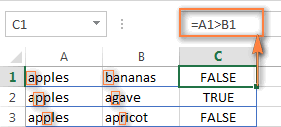At first sight, the use of comparison operators with text values seems to have very little practical sense, but you never know what you might need in the future, so probably this knowledge will prove helpful to someone.

## Common uses of logical operators in Excel

In real work, Excel logical operators are rarely used on their own. Agree, the Boolean values TRUE and FALSE they return, though very true (excuse the pun), are not very meaningful. To get more sensible results, you can use logical operators as part of Excel functions or conditional formatting rules, as demonstrated in the below examples.

#### 1. Using logical operators in arguments of Excel functions

When it comes to logical operators, Excel is very permissive and allows using them in parameters of many functions. One of the most common uses is found in Excel IF function where the comparison operators can help to construct a logical test, and the IF formula will return an appropriate result depending on whether the test evaluates to TRUE or FALSE. For example:

`=IF(A1>=B1, "OK", "Not OK")`

This simple IF formula returns OK if a value in cell A1 is greater than or equal to a value in cell B1, "Not OK" otherwise.

And here's another example:

`=IF(A1<>B1, SUM(A1:C1), "")`

The formula compares the values in cells A1 and B1, and if A1 is not equal to B1, the sum of values in cells A1:C1 is returned, an empty string otherwise.

Excel logical operators are also widely used in special IF functions such as SUMIF, COUNTIF, AVERAGEIF and their plural counterparts that return a result based on a certain condition or multiple conditions.

You can find a wealth of formula examples in the following tutorials:

#### 2. Using Excel logical operators in mathematical calculations

Of course, Excel functions are very powerful, but you don't always have to use them to achieve the desired result. For example, the results returned by the following two formulas are identical:

IF function: `=IF(B2>C2, B2*10, B2*5)`

Formula with logical operators: `=(B2>C2)*(B2*10)+(B2<=C2)*(B2*5)`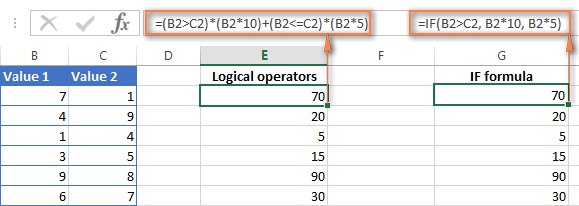I guess the IF formula is easier to interpret, right? It tells Excel to multiply a value in cell B2 by 10 if B2 is greater than C2, otherwise the value in B1 is multiplied by 5.

Now, let's analyze what the 2nd formula with the greater than and less than or equal to logical operators does. It helps to know that in mathematical calculations Excel does equate the Boolean value TRUE to 1, and FALSE to 0. Keeping this in mind, let's see what each of the logical expressions actually returns.

If a value in cell B2 is greater than a value in C2, then the expression B2>C2 is TRUE, and consequently equal to 1. On the other hand, B2<=C2 is FALSE and equal to 0. So, given that B2>C2, our formula undergoes the following transformation: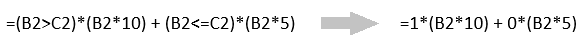Since any number multiplied by zero gives zero, we can cast away the second part of the formula after the plus sign. And because any number multiplied by 1 is that number, our complex formula turns into a simple =B2*10 that returns the product of multiplying B2 by 10, which is exactly what the above IF formula does : )

Obviously, if a value in cell B2 is less than in C2, then the expression B2>C2 evaluates to FALSE (0) and B2<=C2 to TRUE (1), meaning that the reverse of the described above will occur.

#### 3. Logical operators in Excel conditional formatting

Another common use of logical operators is found in Excel Conditional Formatting that lets you quickly highlight the most important information in a spreadsheet.

For example, the following simple rules highlight selected cells or entire rows in your worksheet depending on a value in column A:

Less than (orange): `=A1<5`

Greater than (green): `=A1>20`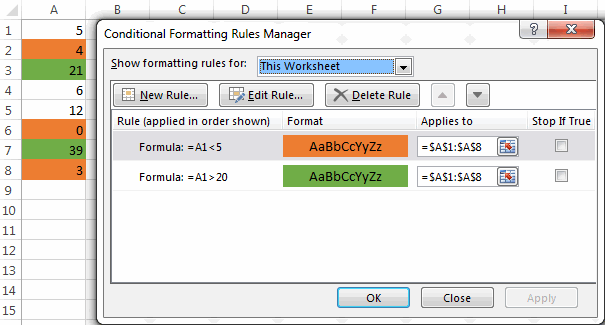For the detailed-step-by-step instructions and rule examples, please see the following articles:

As you see, the use of logical operators in Excel is intuitive and easy. In the next article, we are going to learn the nuts and bolts of Excel logical functions that allow performing more than one comparison in a formula. Please stay tuned and thank you for reading!

## You may also be interested in

1. If I have 3 column of numbers how can I add a 4th with an up, down, or side arrow depending on where the highest number is. Column A has the number of negative reviews recieved, column b has number of neutral reviews received, column c has number of positive reviews received.

For example:

Column A = Down arrow
Column B = Side arrow
Column C = up Arrow

5 9 1 = Side arrow
9 5 1 = Down arrow
1 5 9 = Up arrow

• Hello!
Use the MATCH and MAX functions to find the position of the maximum number. The CHOOSE function selects the desired symbol.

=CHOOSE(MATCH(MAX(A1:C1),A1:C1,0),"↓","→","↑")

2. Hi,

What formula should I use to make a categorized column?
I'm trying to create a column using the contents from my Year_Birth column.
Ex: >=1946 but also <= 1964, using three more statements for the other generations.

Thank you!

3. I have following integers in this pattern below:

A B C
1 -68 -75 120
2 50 60 -90
How do i make use of formatting frmula to return "1" if two of the cells in A1, B1, C1 are less than zero. AND return "2" if one cell in A2, B2, C2 is less than zero.

Thank you.

• Hi!
You can count the number of cells that are greater than 0. To do this, use the COUNTIF function.
Try the following formula:

=COUNTIF(A1:C1,">0")

• Hello,

One question, struggling with one assignment, searched everywhere for a solution but I couldn't find it.
We have 2 columns with A1 name and B2 score and we need to assign to the C3 Grade the third column the result.
In case that the score is equal to 50 or smaller than 50 the result will be Bad
If score is between 51-80 or is equal to 51-80 normal
If score is between 91-110 or is equal to 91-110 best
51-90 Normal
91-110 Best

4. Hi, I'm rather new to excel and I'm wondering whether it would be possible to change the colour of the cell depending on if it was greater than or less than by a certain value so for instance:

Column A Column B
1 11 15
2 11 9

So, it would be something like if B1 is greather than A1 by 3 then green but if less than by 2 then red.
Apologies for the trouble and if not appropriate!

5. Hi

I am looking for a formula that I can use to determine True or False (for conditional formatting prepose).

I want to be able to format a cell containing a value that is greater but not equal to a value in a different cell... So Greater (>) but not equal () to the reference value in a separate formula or cell.

6. Hi,

Bit of a long story but here goes...

I have 2 columns with differing dates across 2021, 2022 and 2023, and I have set up 2 other columns to extract just the month and year - =IF(F2="","",MONTH(F2)&"/"&YEAR(F2)) & =IF(G2="","",MONTH(G2)&"/"&YEAR(G2)) - this is used to compare changes from column F and G (F being old date and G being a new updated date).

I now need to create a countifs to count a specific group of cells with the following criteria:

=COUNTIFS('Tracker'!\$I:\$I,"9/2022",'Tracker'!\$H:\$H,"9/2022",'Tracker'!\$M:\$M,"Yes")

however I do not get the results I expect when I count manually and I believe it's the "9/2022" part causing the issue. I have successfully used countifs reducing it to "9" (as in just the month) however I need to include the year too as to only search the specific September.

Hope that all makes sense and you can help!

C

• For whatever reason the not equal to signs weren't included in the post!

7. Hi everybody,

Does there exist a Formula whereby everything I write in a Cell=1, for example:
C3 has the text O00000001
C4 has the text O00000002
C5 has the text O00000003, and so on.

The Formula that I have in place is: =(C3:C300)*27.50. However, in this case the value on C3:C300 has to be numerical, otherwise it doesn't add it up. In my case these are order IDs (starting with O-Oscar)

So, let's say I have 97 cells in C column written with that sequence (O0000001-O0000300)

I have 6 other columns and it would be very helpful if I can simply write:
C3:O00001 D5: O000045 F9: O0004523

Whereby CDF and other have of course different values.

My question, simply put...

Is there a way to make excel count my Order ID on any of the cells as a simple "1".
I tried with IF, didn't work, or I didnt know the exact formula.

Thanks

8. Good day! I have a formula in excel which is =IF(AJ13>=92,1, IF(AJ13>=88,1.25, IF(AJ13>=84,1.5, IF(AJ13>=80,1.75, IF(AJ13>=76,2, IF(AJ13>=72,2.25, IF(AJ13>=68,2.5, IF(AJ13>=64,2.75, IF(AJ13>=60,3,5)))))))))
My problem is, if the data is 88, the result is 1.5 but as you can see I have clearly written in the formula if the data is greater than or equal to 88, the result should be 1.25. Can you help me with this issue? I'll appreciate it a lot. Thanks!

• Hi!
I was unable to replicate your issue. If AJ13=88 then the formula returns 1.25
If in cell AJ13 the result is obtained using a formula, then use rounding the number to an integer.

• Thanks for replying to my question! I'll try to do your suggestion. Thanks again!

9. Is this correct?
1+21=22 i need formula of round off to one digit 22 = round off to 20
2+34=36 this is round off to= 40

10. hELLO
I have written

=COUNTIFS(D7:D9,">="&AC10,D7:D9,"="&AC10 getting "VALUE!
NEXT
D7:D9 getting a number
"<="&AC10 getting "VALUE!

Can you fix it?
Thank you

• mayby this is the right one.

• I HAVE EXCEL 2000

Thank you

• The post Does not pass properly.
Can you send me an email where I can send properly?

• Thank you

11. I have a value in cell "A1" (80). A value in cell "B1" (70). Cell "C1" has a formula (=B1-A1) which yields "-10" in "C1".

Cell "D1" has a formula: if(C1<=-10,"yes","")

It works and I get a "yes" if the value in "C1" is less than -10 but I get a blank ("") if the value EQUALS "C1".
If I type in "-10" into cell "D1" it recognizes the "=" part of the function and it works.

I tried substituting "≤" for "<=" to no avail.

• Hello!
Your values in A1 and B1 are not integers. Perhaps they are obtained using formulas. Use the ROUND or INT or other rounding function as described in this instruction: How to round numbers in Excel.
I hope this will help.

12. Hi..
The formula in column C is IF(A1=B1,1,0)

B B 1
C C 1
B B 1
A A 0
C C 1
A D 0
C B 0
A A 0
D D 0
D D 0
B B 1
A D 0

Why it is returning zero for equal values... like D D should return 1

• Hi!
Check the values in the cells. Perhaps they contain extra spaces or non-printing characters

13. What formulae do i use to get the performance status as per below matrix

% Achieved Market Performance Status
100% Top Performer
Above 90% Satisfactory Performer
Below 90% Unsatisfactory Performer

• Hi!
Explain in detail what result you want to get.

14. Hello I have a formula in mind, but I can't write it down in excel maybe you can help me with it.
We have a range between 500 and 600, if a result is lower than 500 then its equal 0, but if its higher or equal than 500 we multiply the result by 2. But maximum result be multiplied by 2 can be up to 600. So if we have a result 700, only 600 will be multiplied by 2.

Do you know what I mean?

15. what formula do I use for table type fee schedule like values greater than 0 but not less than or equal to 500 and get 30 for its answer? please help me

16. How to compare. ...true r false....example

F1. G1. Diff

Timestamp. TIME_STAMP

17. Hi! What formula can be used for:

- Let's say the salary is in column G55.
- So, G55 > \$1,600 but < \$1,500...contribution should detected as \$6.20.

• Thank you! The article was a great help!!

18. Need one help

Example:

I have INR 400 .

I need following logic

1St INR 200 should be allocated in 35%
1St INR 80 should be allocated in 30%
and balance to be allocated in 35%

19. Hi,

I want to achieve the following please:
cell D1 and E1 have different dates and the result of the dates is in cell F1 with Today() function.
cell G1 have a text "Done", whenever type "Done" in G1, it should reflect to cell F1 by ignoring whatever in cell F1.

• Hi!
From your description it is very difficult to understand what is written in cell F1. But you can completely replace the contents of this cell, ignoring everything in it, only with the help of a VBA macro.
We have already written many times that an Excel formula can only change the cell in which it is written.

• CELL"D" CELL"E" CELL"F" =E1-TODAY() CELL"G"
17/01/2022 25/04/2022 67 Days Done
20/01/2022 09/03/2022 20 Days

above example,
Cell F have the difference of Cell E and Today() in days, so when I type "Done" in Cell G,
Cell F values (67Days) to be replaced as "Done"

• If I understand your task correctly, the following formula with IF function should work for you:

=IF(G1="Done","Done",E1-TODAY())

• Thank you - That's what I want - Excellent

Thanks a million.

20. Hello all,

I really need urgent help with regards to if operator. I have some dates in column A and other in column B. I want to keep the maximum of both the dates. So I use the following code: IF (A1>B1, A1,B1). While this works beautifully when we dont have blanks or hyphens. But in some case where a cell is having a hyphen it gives me a hyphen again in my new generated column, which I dont want, I want whatever available year is there in either column A or column B it should come out in my new generated column.

A B C [if A>=B, A,B)
1990 1995 1995
2000 - - [I want this to be as 2000]

What is problematic when I go for a <= code in the same shown example then I do get whatever the available year is. By this I mean the following:

A B C [if A<=B, A,B)
1990 1995 1990
2000 - 2000[I want this to be as 2000]

Why this discrepancy is happening, where am I going wrong here? Is something there that I am missing out?

P.S. Since my analysis has some other connected codes in the same column, I cannot delete hyphens and keep them as blanks. And neither I can use a max code because max gives you 0 in case both the columns A and B have blanks which again is not desirable for me.

• Hello!
You are comparing a number with a character and therefore get the wrong result. Use the ISNUMBER function to test a value for a number.

=IF(IF(ISNUMBER(A1),A1,0)>IF(ISNUMBER(B1),B1,0),A1,B1)

21. Hi Alexander,

In the first tab I have a list of words "orange" Cell A1 , "banana" CellA2, "pear" cell A3 etc. In the second tab I have a number of other words "ba" CellA1, "ae" CellA2, "xg" Cell A3 etc. I need to write a formula to compare each word in the first tab against all the words in the second tab and if there is a match to any of the words, to return True/False,

For example,
A1 Orange = False (not matches to anything in the second tab)
A2 Banana = TRUE (matches to 'ba' in cell A1 - second tab)
A3 Pear = TRUE (matches to 'ea' in the Cell A2 - second tab)

What formula do you think I can use to do that?

Thank you!

• Hello!
If I understand your task correctly, the following formula should work for you:

=IF(SUM(IFERROR(SEARCH(Sheet1!A1,Sheet2!\$A\$1:\$A\$10)*(Sheet2!\$A\$1:\$A\$10<>""),0)) + SUM(IFERROR(SEARCH(Sheet2!\$A\$1:\$A\$10,Sheet1!A1)*(Sheet2!\$A\$1:\$A\$10<>""),0)) > 0,TRUE,FALSE)

• Thank you! This is amazing, it worked!

22. Assume that there are 3 independent columns in excel with both plus and negative values. The first two columns need to be added and the sign (positive or negative) of the result is noted. Also, note the sign of the 3rd column value on the same row. Then both signs should be compared. If both signs are the same then print “c” on the 4th column. If the signs are different print “w” on the 4th column. Take the 3rd column zero value as a negative value in case you get a zero on the 3rd column. Thanks for your help.

• Hi!

=IF((A1+B1)*IF(C1=0,-1,C1) > 0,"C","W")

23. IF MY PARAMETERS ARE: IF A SQUAR IS(<than 1M IN WIDTH and < than 25M IN LENTH and <than100M2 in squares( IT WILL BE "patching" otherwise it will be "milling"

What would be the formula

24. I have 4 values in different cells MP-50, UP-20,HP-40 AND AP-6 . how to compare a cell value is less than or equal to this value . If a cell contain MP-7 than pls give me formula to solve this

• Hi!
How do you want to compare text values? What result do you want to get exactly?

25. Description A/C Description A/C
Opening Balance Opening Balance Assets
Rolling Chair Rolling Chair Assets
Color & Brush Color & Brush Assets
Steel Nail Steel Nail Assets

I want to copy A/C as entering description in first column and it auto collect A/C from last column

• Hi!
I don't really understand what you want to do. Perhaps you should pay attention to the VLOOKUP function.

26. Dear sir,
kindly help to set formula in excel for to compare two value which is greater than the grater value will be multiple to other value in between two value

• Hi!

• Dear sir,
if Cell A1 and B1 is comparing with these two which is a greater value in between A1 and B1 to multiple with other value or cell example A1B1 whichever is greater the greater cell is multiple with C1

• Hi!
I asked you to write an example. This means using numbers and writing in which cells they are written. What are you comparing A1 and B1 to? What is A1B1?

• Example ( A:1 100 box, B:1 50 box, if i want to take in this which is greater in these two to price for boxes which one is higher box quantity to multiple with a reasonable amount to the supplier suppose
if A:1 have 100 box B:1 have 50 box
greater of these two A:1><B:1 to detect automatically which one is greater and then to multiple as per price

• Hi!
I’m sorry but your description doesn’t give me a complete understanding of your task. However, I’ll try to guess and offer you the following formula:

=IF(A1>B1,G1*C1,G1*D1)

• Thanks a lot, Sir its work and it help me your genius thank you very much sir very very thanks

27. my D column has two options: Apple, Orange
my E column is the dispense
My F column is the due date; based on type and dispense date

Question:
If D says apple then my F is 21 days from E
If D says orange then my F is 28 days from E

How do I format my due date?

• I'm making a computation for a crypto game, what is the proper formula for this? I want to leave my battle result column blank but it's still showing "Lose". Can anyone help me with this? Thanks in advance!

=IF(E8>3,"Win",IF(E8<3,"Lose",IF(OR(E8=0,ISBLANK(E8))," ")))

This is the formula that I used.

• Hello!

=IF(E8>3,"Win",IF(OR(E8=0,ISBLANK(E8))," ","Lose"))

• Hi Alexander! I tried the formula above and it worked! I truly appreciate it!

28. Hello, I have a specific need which I have not been able to work out through all my trials and searching. I need the fill color of A5 to be yellow if C5 is 240 greater than B5 but less than 351 more than B5. And also need A5 fill color to be red if C5 is greater than 350 more than B5. Is there a formula for this? Example: A5 is yellow fill when B5=2210 and C5=2505, and A5 is red fill when B5=1965 and C5=2475. Is there a formula out there for this? I appreciate any help to come my way.

• Hello!
Create the first conditional formatting rule with condition =C5-B5>240 and the second rule with condition =C5-B5>350. Set the priority of the second rule as described in this guide: Apply several conditional formatting rules to one cell.

29. why this is not working in case of custom data validation =OR((F5"Book1"),(F5"Book2"),(F5"Book3"))

30. i am not sure how to do it

If the value in A1 is greater than or equal to 250 but not equal to 400,return 250, and if A1 is greater that or equal to 400 but not equal to 800,return 400, and if the value in A1 is greater than or equal to 800 bit not equal to 2000,return 800.

32. Why oh why does this not work:
IF(AND(\$D\$3="Smith Inc.",\$A\$2=2),C4+D4,C4)

• I'm not sure what's happening when i post my comment, but it's removing the "not equal symbols " The formula should read IF(AND(D3 "does not equal Smith Inc.",A2=2),C4+D4,C\$)

• Hello!
I don’t know your data source, so I can’t help. Please describe your problem in more detail. It’ll help me understand it better and find a solution for you.

I am wanting one cell in the sheet to count the number of times each status is chosen; status was created as "text from drop down list; created list with data validation rule.", the listed choices are: "In Progress", "On Track", "Stalled", "Overdue", "Not Started", and "Complete".

On my reporting sheet, I have created a cell labeled as "Project Status". In this cell, I need a formula that can calculate how many times each status from the list is chosen and the average of those instances. Finally, I want it to calculate the averaged information as an over all status for the project.

• Hello!
Use the COUNTIF function to calculate how many times each status is applied.
Use the AVERAGEIF function to calculate the average of a condition.

• Much appreciated. I will give that a shot.

34. I have a table and I want to do advanced filtering to find persons with name only beginning with the letter "B" how would I do this?

35. Please help with the formula to be used in counting the number of Students with the range of 75 - 79.

That is the data. Thanks, please

83
83
83
83
83
83
83
77
81
82
75
75
89
89
79
75
79
79
81
91
85
77
76
80
90
88
79
90
80

• Hello!
You can use array formula

=FREQUENCY(A1:A29,{75,79})

Press Ctrl + Shift + Enter so that array function to work.

It will return 3 values:
The first is the count of numbers is less than or equal to 75
Second, the count of numbers is greater than 75 and less than or equal to 79.
Third - more than 79

36. Can you explain how Excel might be able to perform the following function?

A 'true' cell contains the 24 characters A,B,C,D,E,F,G,H,I,J,K,L,1,2,3,4,5,6,7,8,9,10,11,12. A 'false' cell contains duplicated and omitted characters - such as cell A,A,C,D,E,F,G,H,I,J,K,L,1,1,3,4,5,6,7,8,9,10,11,12.

However, if this false cell A,A,C,D,E,F,G,H,I,J,K,L,1,1,3,4,5,6,7,8,9,10,11,12 is cancelled out by another false cell B,B,C,D,E,F,G,H,I,J,K,L,2,2,3,4,5,6,7,8,9,10,11,12, then these two cells are collectively true because every character occurs the same number of times (twice in this example).

The project I'm working on involves large numbers of these false cells produced by a fairly random process. Can Excel identify sets of complementary cells that are collectively true? (these sets could be pairs - as in the above example - triplets, quadruplets, or more. Note that the whole list needn't be collectively true...just that collectively true sets of cells can be located by searches.)

• Hello!
If I understood the problem correctly, your task requires a very large number of comparisons. Even if there are 5 cells, the number of possible combinations is 120. This is a complex solution that cannot be found with a single formula. If you have a specific question about the operation of a function or formula, I will try to answer it.

37. I am trying to get a formula to work to pull a price from a different page based on a number on my main page. ex. if the number is greater than 0 but less than 5 it is one price but if it greater than 5 and less than 10 it is another. Any suggestions?

38. Hi,

I have the following censored datasets reported during microbiological assay -
< 1
< 4
< 10
< 6
< 5
< 4

Is it possible to determine the mean and SD of such numbers? If yes, how? And how can such numbers be further statistically analyzed?

39. Hi.
I am doing the lateness attendance record. We have 2 shift, morning and evening. For example, those who comes above 0706 and 1906 is late.
If I need to put it into formula, how do I do it?
I was thinking to do =if(A2>0705,"L") but how do I combine it with evening shift.

• Hello!
The information you provided is not enough to understand your case and give you any advice, sorry.In what format is your time recorded? In text format like number or time format? Give an example of the source data and the expected result.
It’ll help me understand it better and find a solution for you.

If the value in A1 is greater than or equal to 250 but not equal to 400,return 250, and if A1 is greater that or equal to 400 but not equal to 800,return 400, and if the value in A1 is greater than or equal to 800 bit not equal to 2000,return 800

40. Use a formula to define the Students Marks Grade as given below in Column G.
>=80 is "A", >=60 to ==35 to =<59 is "C", =<34 is "Not Eligible".

41. plz check where is incorect

=IF(AND(L5>80,L585,L590,L595,L5100),I5*100%,0)))))

• =IF(AND(L5>80,L585,L590,L595,L5100),I5*100%,0)))))

42. I have a cell that needs to auto calculate an amount if a 25% is not achieved to make the cell equal to 25. Is there a way for excel to auto calculate that value?

Any help on this would be greatly appreciated.

• Hello!
Sorry, it's not quite clear what you are trying to achieve. From what date do you calculate 25%? Do you want to calculate the sum or set the value to 25? Please describe your problem in more detail. Include an example of the source data and the result you want to get. It’ll help me understand your request better and find a solution for you.

43. Hi everyone, need help on this problem.

A1 = Karen (Name of Tenant)
B1 = Total Monthly Rental Payment
C1 = Rental Rate per month - 10,000.00
E1 = Less EWT 5%
F1 = PEZA accredited

i want that result on B1 should be non-VAT 12% exclusive if the tenant is PEZA accredited

pls. help me on my current formula

current formula: =(I5+G5)-H5

44. Hi there,

I have some excel question and below is some examples of the question.

I have a pallet and I would like to arrange my box on the this pallet. My Box stacking is not exceed more than 5 feet.

Pallet size is 1100x110x130 in mm.
Box size is 406x345x258 in mm

Base on size dimension above, manual calculation is as below

X : 1100 / 406 = 2.709 (this means only 2 box I only able to arrange in X direction)
Y : 1100 /345 = 3.188 (this means only 3 box I only able to arrange in Y direction)
Z :[ (5ft x 12 x 25.4) - 130] / 258 = 5.403 (this means only able to arrange the Box in 5 layer, Z direction)

Total Box in one pallet is = 2 x 3 x 5
= 30 boxes / pallet

The question is how can I change the answer 2.709 become 2 using excel equation?

45. Formula to return cell (eg A21 value) if value lies between >X<Y where X&Y are cell values. Please! Thanks

46. I have huge data with different Values and have to derive at some classification based on three data sets.

Col A & B has Numbers as Values and Col C has Names as Values

Col A has Values ranging from 0 to 1000
Col B has Values ranging from 0 to 1000000
Col C has Values WHITE, RED, ORANGE, BLUE

Could anyone help me to get this please. Below is an example of what I am looking for.

if A is greater than 1 and equals to 4 and is WHITE or RED term it as Medium
if B is greater than 8999 and equals to 16999 term it as Medium

47. I have a formula that I am stuck building It is using the below information. Can you please advise how to build the "if then" formula cell C5 for the below? I am stuck on trying to get the in between numbers to work. so far I have gotten = IF(C5<4,5,(C5

5 = 0 to 4
4 = 4.01 to 12
3 = 12.01 to 24
2 = 24.01 to 28
1 = 28<

48. I HAVE THREE SHEET, WITH SAME HEADER BUT VARIOUS VALUE IN IT, HOW TO KNOW WHICH SHEET DATA LESS THEN OUT OF THREE. CAN ANY OPTION OR COMMAND TO PRINT LESS SHEET NAME

SHEET NAME
1. 3338 DATA A 1620
2. 5498 DATA A 1550
3. 5913 DATA A 1820

49. 1+21=22 i need formula of round off one digit 22 = round on 20
2+34=36 this is round on = 40

• you can use Mround function.

50. Hi,

What formula should I use for:
If the value is not equal to "follow" in C4 then get the value in C4.

Thanks!

• Hello!